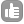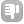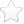0Hello, I am the first time confronted with a 13C-NMR, in which a coupling between the 13C and 31-P cores takes place. I am very unsure about the signal assignment in the molecule. Here you can see the molecule: link:molecule I have assigned the signals so that the coupling becomes smaller at a greater distance from the 31P core. My "Assignment": 13C NMR (101 MHz, CDCl3): ? (ppm) = 232.64 (d, J = 25.2 Hz, C (14.15)), 138.32 (d, J = 40.3 Hz, C (8)), 133.07 (i.e. , J = 9.9Hz, C (9.13)), 129.59 (C (10.12)), 128.23 (d, J = 8.9Hz, 3.4)), 87.57 (C (2.5)), 80.98 (C (1)), -3.10 (C (6.7)). However, I am very unsure about the assignment of the 13C signals of the cyclopentadienyl ring. I would like to understand the correct assignment correctly. I am very grateful for every help as well as for every suggestion! best regards Sabine <3 asked Oct 21 '17 at 13:32Be the first one to answer this question!